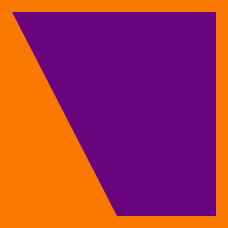Geometry

#### Challenge Quizzes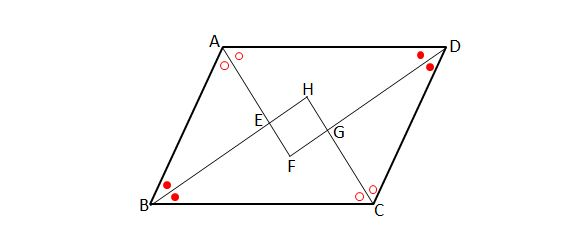Figure $$ABCD$$ is a parallelogram with $$\lvert{\overline{AB}} \rvert =7$$ and $$\lvert{\overline{AD}}\rvert=10.$$ If the points $$E,F,G$$ and $$H$$ are intersections between the angular bisectors of the four internal angles of the the parallelogram, what type of quadrilateral is $$EFGH$$?

Note: The above diagram is not drawn to scale.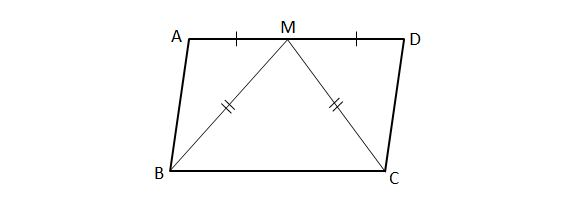$$ABCD$$ is a parallelogram and point $$M$$ is the midpoint of side $$\overline{AD}$$ where $\lvert{\overline{AB}}\rvert=10, \lvert{\overline{AD}}\rvert=12.$ If $$\lvert{\overline{BM}}\rvert=\lvert{\overline{CM}}\rvert,$$ what type of quadrilateral is $$ABCD$$?

Note: The above diagram is not drawn to scale.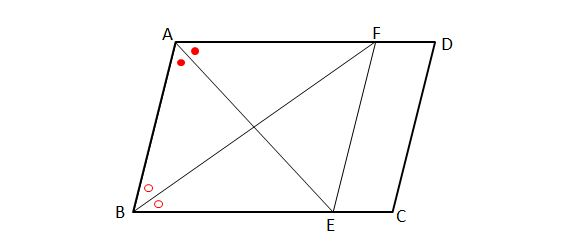In the above diagram, figure $$ABCD$$ is a parallelogram where $$\lvert{\overline{AB}} \rvert =6$$ and $$\lvert{\overline{AD}}\rvert=8.$$ If the angle bisectors of $$\angle A$$ and $$\angle B$$ intersect with $$\overline{BC}$$ and $$\overline{AD}$$ at $$E$$ and $$F,$$ respectively, which of the following must be true of quadrilateral $$ABEF ?$$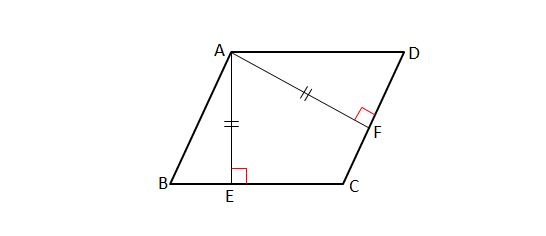In the above diagram, points $$E$$ and $$F$$ are the feet of perpendiculars drawn from vertex $$A$$ of parallelogram $$ABCD$$ to sides $$\overline{BC}$$ and $$\overline{CD},$$ respectively. If $\lvert{\overline{AE}}\rvert=\lvert{\overline{AF}}\rvert=13,$ what type of quadrilateral is $$ABCD$$?

Note: The above diagram is not drawn to scale.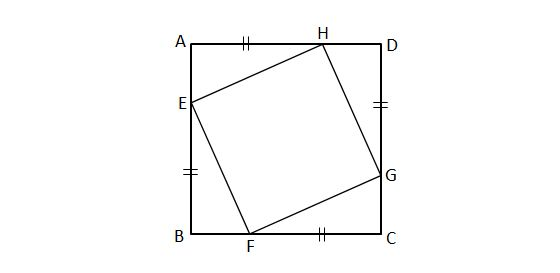Figure $$ABCD$$ is a square with side length $$12$$ and $\lvert{\overline{EB}}\rvert =\lvert{\overline{FC}}\rvert=\lvert{\overline{GD}}\rvert=\lvert{\overline{HA}}\rvert=9.$ What type of quadrilateral is $$EFGH$$?

×